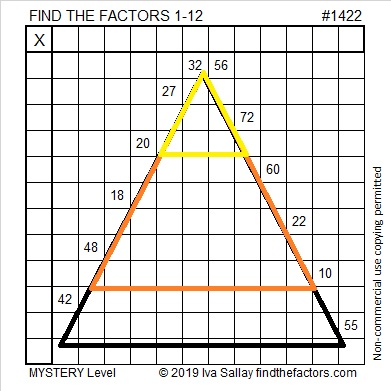# 1422 Candy Corn Mystery

You can begin this candy corn puzzle easily enough, but the logic needed to solve it is a bit complicated. Good luck with this one!Print the puzzles or type the solution in this excel file: 12 Factors 1419-1429

Now I’ll tell you some facts about the puzzle number, 1422:

• 1422 is a composite number.
• Prime factorization: 1422 = 2 × 3 × 3 × 79, which can be written 1422 = 2 × 3² × 79
• 1422 has at least one exponent greater than 1 in its prime factorization so √1422 can be simplified. Taking the factor pair from the factor pair table below with the largest square number factor, we get √1422 = (√9)(√158) = 3√158
• The exponents in the prime factorization are 1, 2, and 1. Adding one to each exponent and multiplying we get (1 + 1)(2 + 1)(1 + 1) = 2 × 3 × 2 = 12. Therefore 1422 has exactly 12 factors.
• The factors of 1422 are outlined with their factor pair partners in the graphic below.1422 is palindrome 1K1 in BASE 29 (K is 20 base 10)
because 1(29²) + 20(29¹) + 1(29º) = 1422.